Mathematics is one such subject which needs thorough practice by students in obtaining a good overall rank in Class 6 exam. The PDF provided contains exercise wise solutions in detail, which helps students to understand effortlessly. Exercise 1(A) gives an idea to students about comparing numbers, numbers in ascending and descending order, effect of shifting digits. The Solutions PDF can be used as a reference guide while solving problems from the textbook. To know more about the concepts which are explained here, students can access Selina Solutions Concise Mathematics Class 6 Chapter 1 Number System Exercise 1(A) PDF, from the links given below

## Selina Solutions Concise Mathematics Class 6 Chapter 1 Number System Exercise 1(A) Download PDF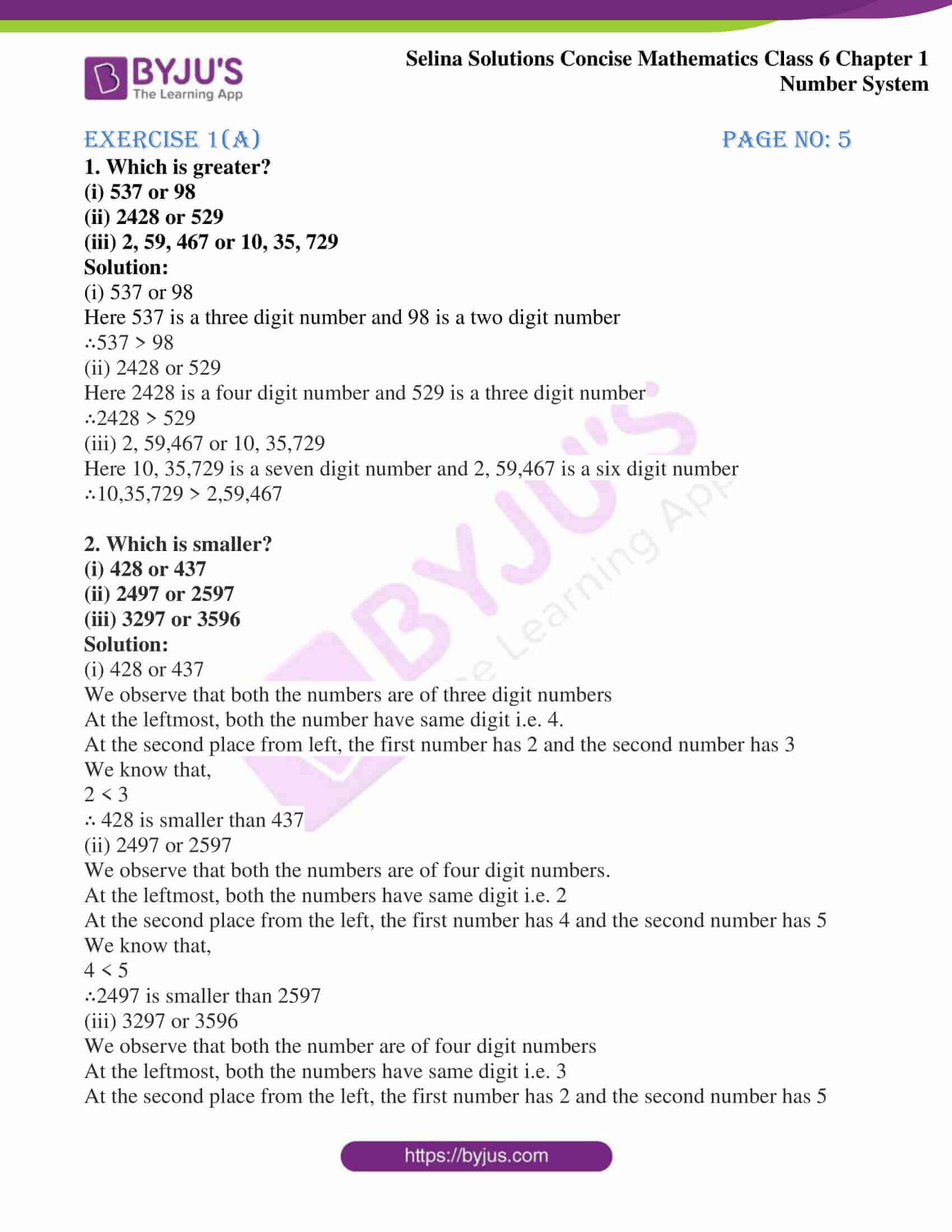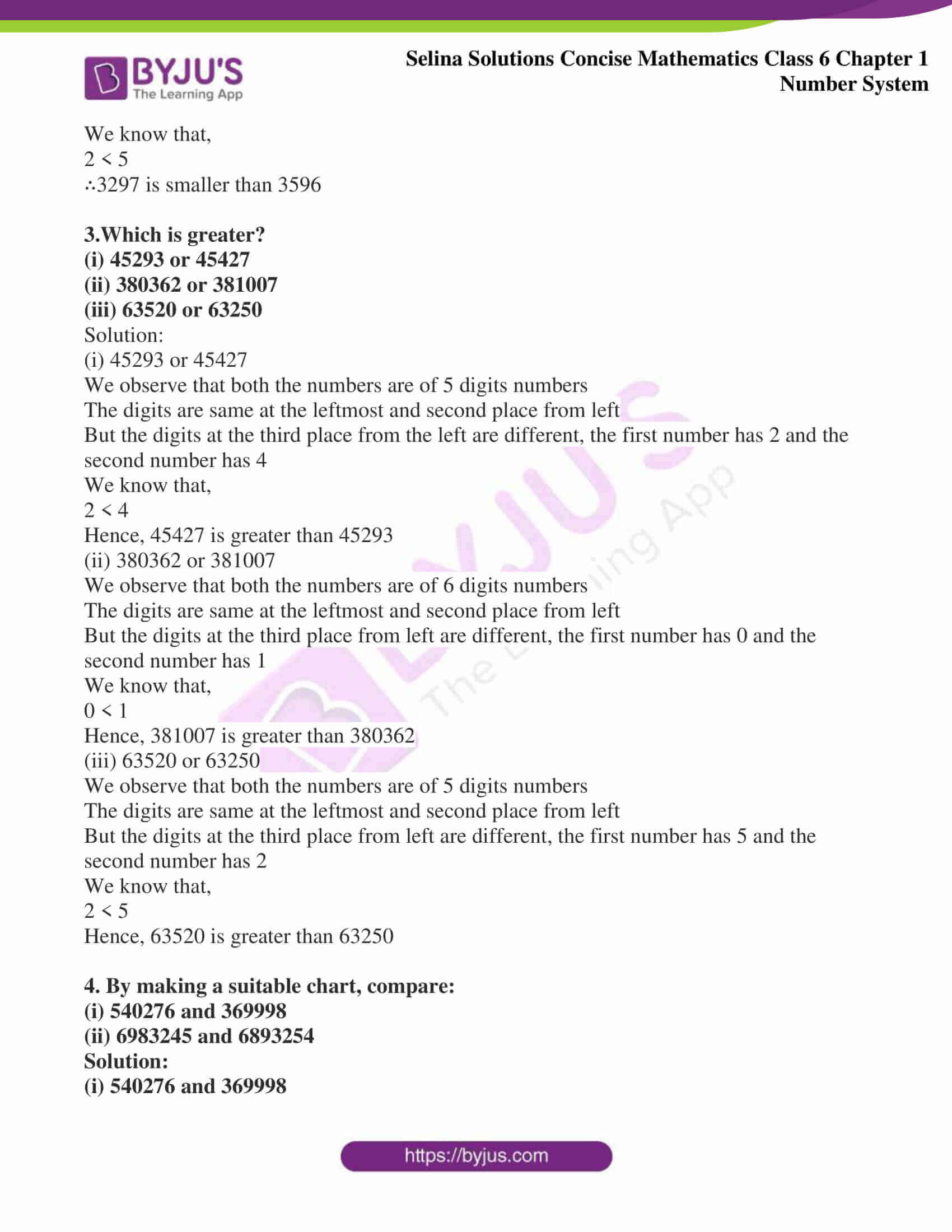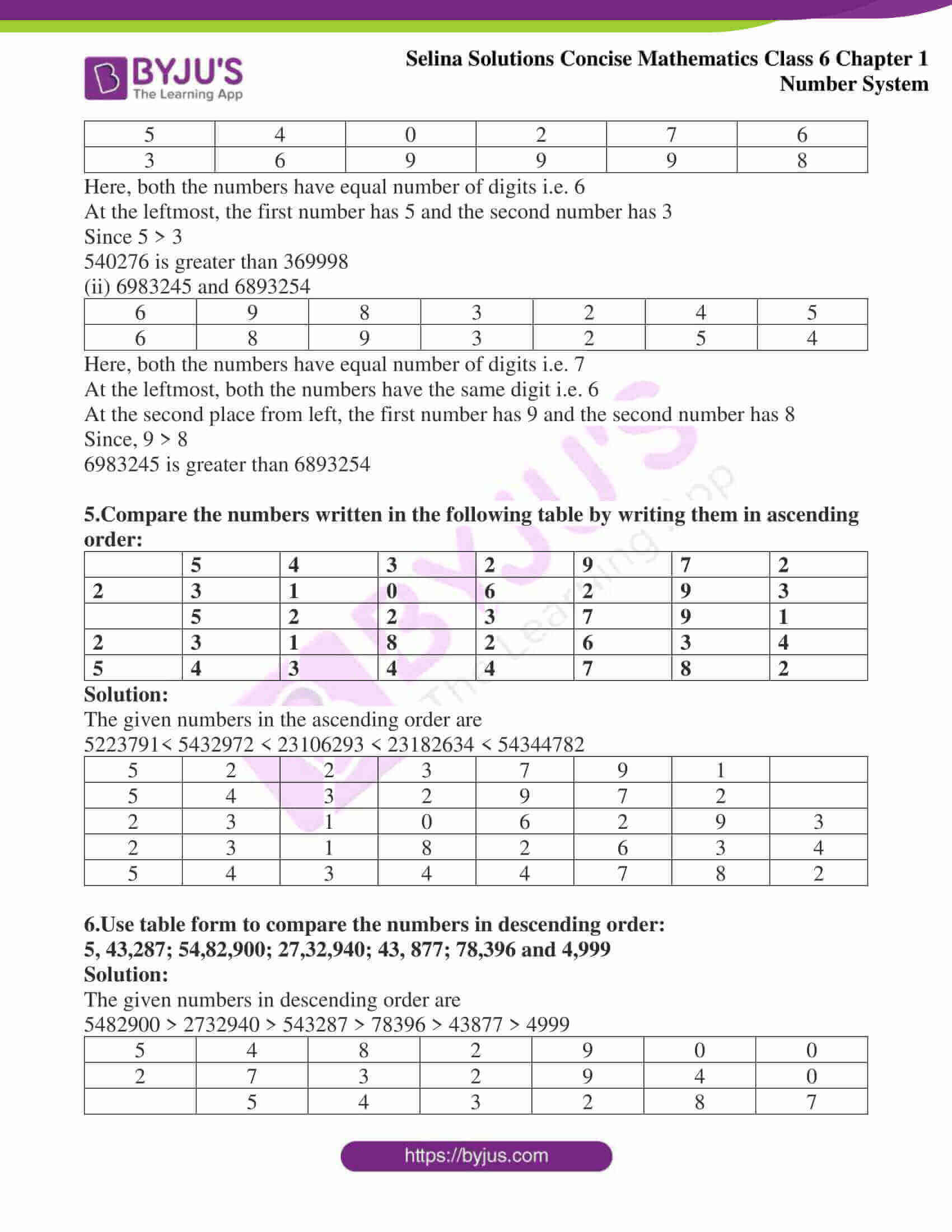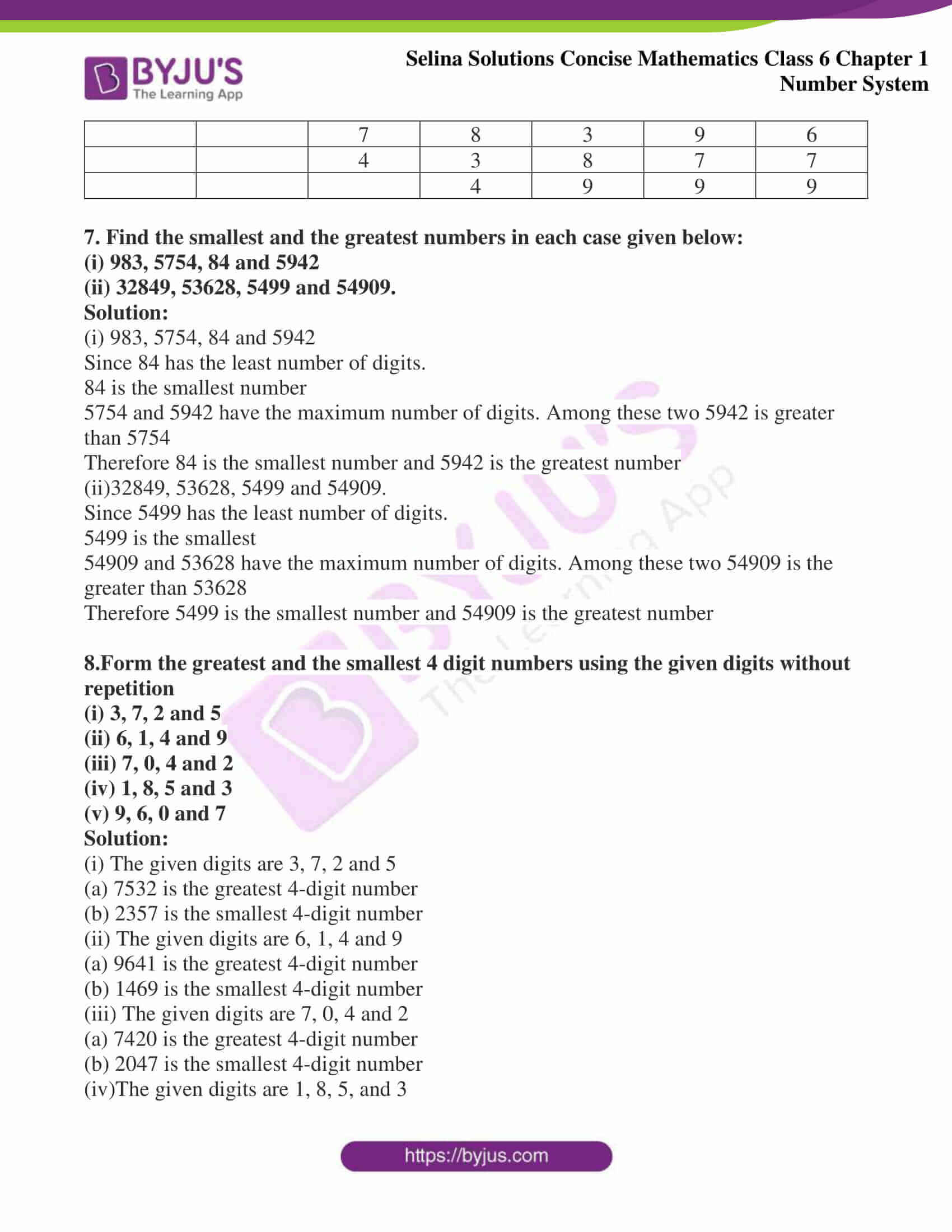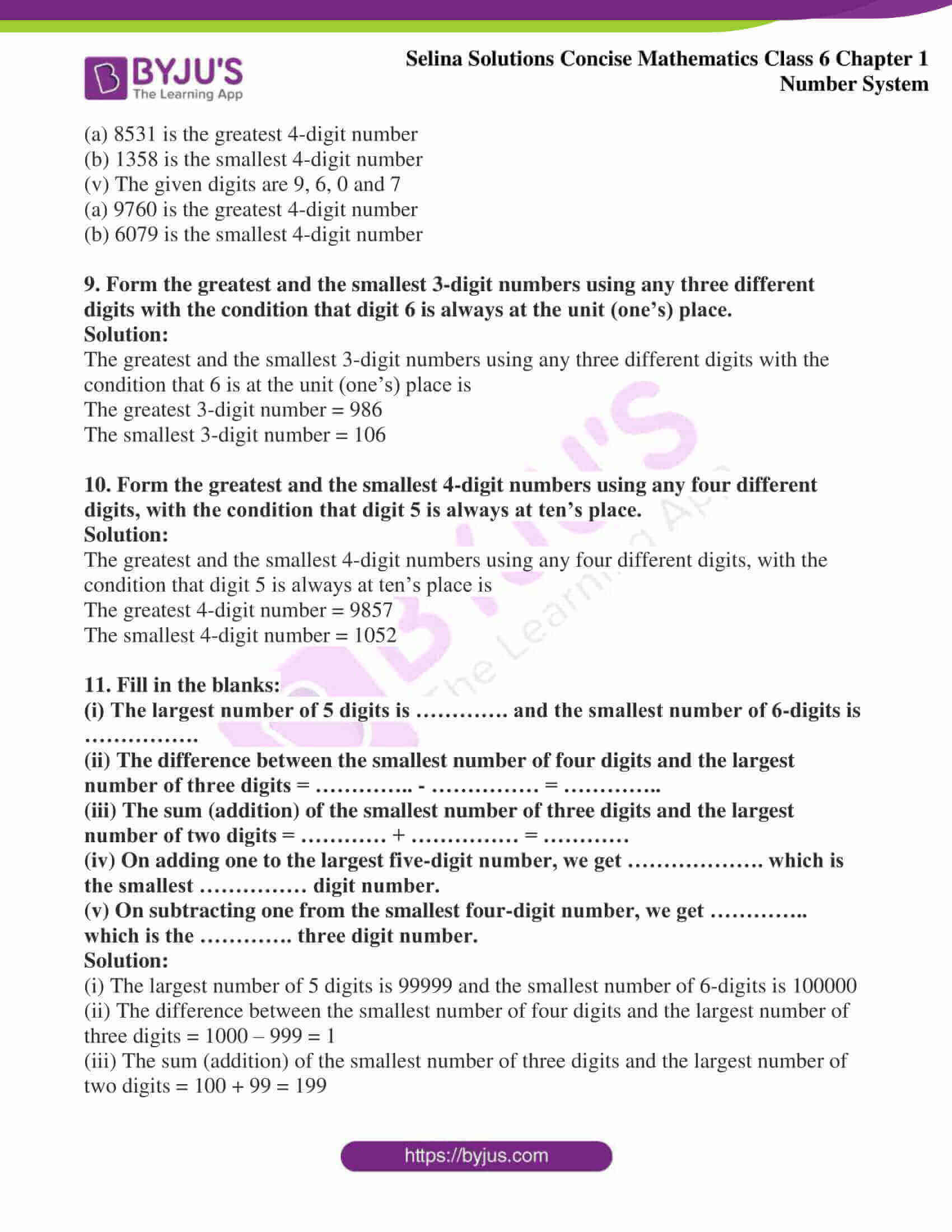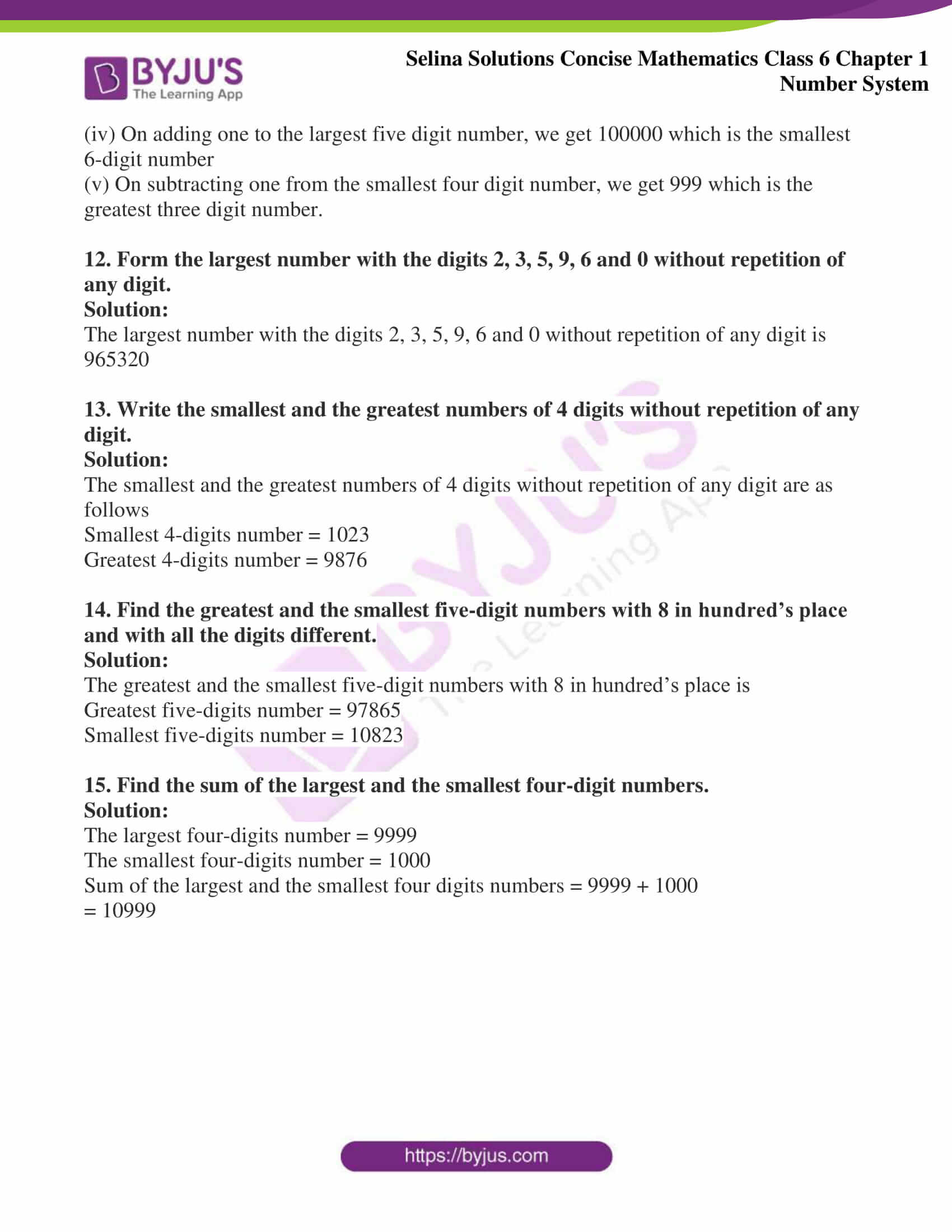### Access answers of Selina Solutions Concise Mathematics Class 6 Chapter 1 Number System

#### Exercise 1(A) Page no: 5

1. Which is greater?
(i) 537 or 98
(ii) 2428 or 529
(iii) 2, 59, 467 or 10, 35, 729

Solution:

(i) 537 or 98

Here 537 is a three digit number and 98 is a two digit number

∴537 > 98

(ii) 2428 or 529

Here 2428 is a four digit number and 529 is a three digit number

∴2428 > 529

(iii) 2, 59,467 or 10, 35,729

Here 10, 35,729 is a seven digit number and 2, 59,467 is a six digit number

∴10,35,729 > 2,59,467

2. Which is smaller?
(i) 428 or 437
(ii) 2497 or 2597
(iii) 3297 or 3596

Solution:

(i) 428 or 437

We observe that both the numbers are of three digit numbers

At the leftmost, both the number have same digit i.e. 4.

At the second place from left, the first number has 2 and the second number has 3

We know that,

2 < 3

∴ 428 is smaller than 437

(ii) 2497 or 2597

We observe that both the numbers are of four digit numbers.

At the leftmost, both the numbers have same digit i.e. 2

At the second place from the left, the first number has 4 and the second number has 5

We know that,

4 < 5

∴2497 is smaller than 2597

(iii) 3297 or 3596

We observe that both the number are of four digit numbers

At the leftmost, both the numbers have same digit i.e. 3

At the second place from the left, the first number has 2 and the second number has 5

We know that,

2 < 5

∴3297 is smaller than 3596

3.Which is greater?
(i) 45293 or 45427
(ii) 380362 or 381007
(iii) 63520 or 63250

Solution:

(i) 45293 or 45427

We observe that both the numbers are of 5 digits numbers

The digits are same at the leftmost and second place from left

But the digits at the third place from the left are different, the first number has 2 and the second number has 4

We know that,

2 < 4

Hence, 45427 is greater than 45293

(ii) 380362 or 381007

We observe that both the numbers are of 6 digits numbers

The digits are same at the leftmost and second place from left

But the digits at the third place from left are different, the first number has 0 and the second number has 1

We know that,

0 < 1

Hence, 381007 is greater than 380362

(iii) 63520 or 63250

We observe that both the numbers are of 5 digits numbers

The digits are same at the leftmost and second place from left

But the digits at the third place from left are different, the first number has 5 and the second number has 2

We know that,

2 < 5

Hence, 63520 is greater than 63250

4. By making a suitable chart, compare:
(i) 540276 and 369998
(ii) 6983245 and 6893254

Solution:

(i) 540276 and 369998

 5 4 0 2 7 6 3 6 9 9 9 8

Here, both the numbers have equal number of digits i.e. 6

At the leftmost, the first number has 5 and the second number has 3

Since 5 > 3

540276 is greater than 369998

(ii) 6983245 and 6893254

 6 9 8 3 2 4 5 6 8 9 3 2 5 4

Here, both the numbers have equal number of digits i.e. 7

At the leftmost, both the numbers have the same digit i.e. 6

At the second place from left, the first number has 9 and the second number has 8

Since, 9 > 8

6983245 is greater than 6893254

5.Compare the numbers written in the following table by writing them in ascending order:

 5 4 3 2 9 7 2 2 3 1 0 6 2 9 3 5 2 2 3 7 9 1 2 3 1 8 2 6 3 4 5 4 3 4 4 7 8 2

Solution:

The given numbers in the ascending order are

5223791< 5432972 < 23106293 < 23182634 < 54344782

 5 2 2 3 7 9 1 5 4 3 2 9 7 2 2 3 1 0 6 2 9 3 2 3 1 8 2 6 3 4 5 4 3 4 4 7 8 2

6.Use table form to compare the numbers in descending order:

5, 43,287; 54,82,900; 27,32,940; 43, 877; 78,396 and 4,999

Solution:

The given numbers in descending order are

5482900 > 2732940 > 543287 > 78396 > 43877 > 4999

 5 4 8 2 9 0 0 2 7 3 2 9 4 0 5 4 3 2 8 7 7 8 3 9 6 4 3 8 7 7 4 9 9 9

7. Find the smallest and the greatest numbers in each case given below:
(i) 983, 5754, 84 and 5942
(ii) 32849, 53628, 5499 and 54909.

Solution:

(i) 983, 5754, 84 and 5942

Since 84 has the least number of digits.

84 is the smallest number

5754 and 5942 have the maximum number of digits. Among these two 5942 is greater than 5754

Therefore 84 is the smallest number and 5942 is the greatest number

(ii)32849, 53628, 5499 and 54909.

Since 5499 has the least number of digits.

5499 is the smallest

54909 and 53628 have the maximum number of digits. Among these two 54909 is the greater than 53628

Therefore 5499 is the smallest number and 54909 is the greatest number

8.Form the greatest and the smallest 4 digit numbers using the given digits without repetition
(i) 3, 7, 2 and 5
(ii) 6, 1, 4 and 9
(iii) 7, 0, 4 and 2
(iv) 1, 8, 5 and 3
(v) 9, 6, 0 and 7

Solution:

(i) The given digits are 3, 7, 2 and 5

(a) 7532 is the greatest 4-digit number

(b) 2357 is the smallest 4-digit number

(ii) The given digits are 6, 1, 4 and 9

(a) 9641 is the greatest 4-digit number

(b) 1469 is the smallest 4-digit number

(iii) The given digits are 7, 0, 4 and 2

(a) 7420 is the greatest 4-digit number

(b) 2047 is the smallest 4-digit number

(iv)The given digits are 1, 8, 5, and 3

(a) 8531 is the greatest 4-digit number

(b) 1358 is the smallest 4-digit number

(v) The given digits are 9, 6, 0 and 7

(a) 9760 is the greatest 4-digit number

(b) 6079 is the smallest 4-digit number

9. Form the greatest and the smallest 3-digit numbers using any three different digits with the condition that digit 6 is always at the unit (one’s) place.

Solution:

The greatest and the smallest 3-digit numbers using any three different digits with the condition that 6 is at the unit (one’s) place is

The greatest 3-digit number = 986

The smallest 3-digit number = 106

10. Form the greatest and the smallest 4-digit numbers using any four different digits, with the condition that digit 5 is always at ten’s place.

Solution:

The greatest and the smallest 4-digit numbers using any four different digits, with the condition that digit 5 is always at ten’s place is

The greatest 4-digit number = 9857

The smallest 4-digit number = 1052

11. Fill in the blanks:

(i) The largest number of 5 digits is …………. and the smallest number of 6-digits is …………….

(ii) The difference between the smallest number of four digits and the largest number of three digits = ………….. – …………… = …………..

(iii) The sum (addition) of the smallest number of three digits and the largest number of two digits = ………… + …………… = …………

(iv) On adding one to the largest five-digit number, we get ………………. which is the smallest …………… digit number.

(v) On subtracting one from the smallest four-digit number, we get ………….. which is the …………. three digit number.

Solution:

(i) The largest number of 5 digits is 99999 and the smallest number of 6-digits is 100000

(ii) The difference between the smallest number of four digits and the largest number of three digits = 1000 – 999 = 1

(iii) The sum (addition) of the smallest number of three digits and the largest number of two digits = 100 + 99 = 199

(iv) On adding one to the largest five digit number, we get 100000 which is the smallest 6-digit number

(v) On subtracting one from the smallest four digit number, we get 999 which is the greatest three digit number.

12. Form the largest number with the digits 2, 3, 5, 9, 6 and 0 without repetition of any digit.

Solution:

The largest number with the digits 2, 3, 5, 9, 6 and 0 without repetition of any digit is 965320

13. Write the smallest and the greatest numbers of 4 digits without repetition of any digit.

Solution:

The smallest and the greatest numbers of 4 digits without repetition of any digit are as follows

Smallest 4-digits number = 1023

Greatest 4-digits number = 9876

14. Find the greatest and the smallest five-digit numbers with 8 in hundred’s place and with all the digits different.

Solution:

The greatest and the smallest five-digit numbers with 8 in hundred’s place is

Greatest five-digits number = 97865

Smallest five-digits number = 10823

15. Find the sum of the largest and the smallest four-digit numbers.

Solution:

The largest four-digits number = 9999

The smallest four-digits number = 1000

Sum of the largest and the smallest four digits numbers = 9999 + 1000

= 10999

## Access another exercise of Selina Solutions Concise Mathematics Class 6 Chapter 1: Number System

Exercise 1(B) Solutions